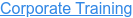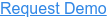by Subashini, on Sep 10, 2022 3:04:32 PM1.What is a multiplexer?
A multiplexer is a combinational circuit which selects one of many input signals and directs to the only output.

2. What is meant by LUT?
LUT – Look-Up Table. An n-bit look-up table can be implemented with a multiplexer whose select lines are the inputs of the LUT and whose inputs are constants. An n-bit LUT can encode any n-input Boolean function by modeling such functions as truth tables. This is an efficient way of encoding Boolean logic functions, and LUTs with 4-6 bits of input are in fact the key component of modern FPGAs.

3.What is meant by bit Binary multiplier
Binary multiplication process: A Binary Multiplier is a digital circuit used in digital electronics to multiply two binary numbers and provide the result as output. The method used to multiply two binary numbers is similar to the method taught to school children for multiplying decimal numbers which is based on calculating partial product, shifting them and adding them together. Similar approach is used to multiply two binary numbers. Long multiplicand is multiplied by 0 or 1 which is much easier than decimal multiplication as product by 0 or 1 is 0 or same number respectively. Figure 1 below shows the block diagram of a 2-bit binary multiplier. The two numbers A1A0 and B1B0 are multiplied together to produce a 4-bit output P3P2P1P0. (The maximum product term can be 3 * 3 = 9, which is 1001, a 4-bit number).

4. What is a ring counter?
A ring counter is a type of counter composed of a circular shift register. The output of the last shift register is fed tothe input of the first register. For example, in a 4-register counter, with initial register values of 1100, the repeatingpattern is: 1100, 0110, 0011, 1001, 1100, so on.

5.What are PLA and PAL? Give the differences between them.
Programmable Logic Array is a programmable device used to implement combinational logic circuits. The PLA has a set of programmable AND planes, which link to a set of programmable OR planes, which can then be conditionally complemented to produce an output.
PAL is programmable array logic, like PLA, it also has a wide, programmable AND plane. Unlike a PLA, the OR plane is fixed, limiting the number of terms that can be ORed together.
Due to fixed OR plane PAL allows extra space, which is used for other basic logic devices, such as multiplexers, exclusive-ORs, and latches. Most importantly, clocked elements, typically flip-flops, could be included in PALs. PALs are also extremely fast.

6.16×1 mux using 4×1 muxes
Implementing 16:1 multiplexer with 4:1 multiplexers: A 16×1 mux can be implemented using 5 4×1 muxes. 4 of these multiplexers can be used as first stage to mux 4 inputs each with two least significant bits of select lines (S0 and S1), resulting in 4 intermediate outputs, which, then can be muxed again using a 4:1 mux. The implementation of 16×1 mux using 4×1 muxes is shown below in figure 1:

7. Compare and Contrast Synchronous and Asynchronous reset.
Synchronous reset logic will synthesize to smaller flip-flops, particularly if the reset is gated with the logicgenerating the d-input. But in such a case, the combinational logic gate count grows, so the overall gate countsavings may not be that significant. The clock works as a filter for small reset glitches; however, if these glitchesoccur near the active clock edge, the Flip-flop could go metastable. In some designs, the reset must be generated bya set of internal conditions. A synchronous reset is recommended for these types of designs because it will filter thelogic equation glitches between clock.Problem with synchronous resets is that the synthesis tool cannot easily distinguish the reset signal from any otherdata signal. Synchronous resets may need a pulse stretcher to guarantee a reset pulse width wide enough to ensurereset is present during an active edge of the clock, if you have a gated clock to save power, the clock may bedisabled coincident with the assertion of reset. Only an asynchronous reset will work in this situation, as the resetmight be removed prior to the resumption of the clock. Designs that are pushing the limit for data path timing, cannot afford to have added gates and additional net delays in the data path due to logic inserted to handle synchronousresets.

8. How can you convert a JK flip-flop to a D flip-flop?
Connect the inverted J input to K input.

9.What is the Reset basics ?
Purpose of reset: We see that almost every electronic device has a reset button. Your video game has a reset button that resets the game and your unsaved progress is lost. Your laptop’s reset button reboots it. Have you ever wondered why a system (or specifically a chip) has a reset? Well, the simple purpose of resets is to provide a known initial state to the system to start with. Another reason is, when the system accidentally goes into some unknown state (there may be many reasons for this), the system always knows how to get out of this and go into a known state by asserting a reset signal.
Reset design strategies
Defining a reset is one of the most important decisions that needs to be taken for the good health of design. In general, following things need to be kept in mind during deciding reset strategy:

• What flops to receive reset: One of the easiest and safest approaches is to enable all the flip-flops in the design with a reset. However, there may be a some registers, whose initial state will not have any impact on the design state. In other words, it might not matter if the register’s output is ‘0’ or ‘1’ when design goes in reset state. Such registers can be kept non-resettable after an analysis. Let us elaborate with the help of an example. Figure 1 shows a part of an FSM wherein two registers are feeding an AND gate. In figure 1(a), we have decided to initialize both to ‘0’ during reset (with an asynchronous reset, to be explained later). However, given this scenario, if one of the flip-flops is provided with an initial state of ‘0’, the output of the other will be gated. So, we may omit reset on one of the flip-flops. Figure 1(b) shows that omission of reset on one of the flip-flops does not have any impact on state machine design.

We may not always encounter such scenarios. If, instead of AND gate, we had an OR gate, we would not have been able to keep one of the flip-flops uninitialized during reset. Figure 2 shows such an example. In figure 2(b), if we remove reset from second flip-flop, output of OR gate goes ‘X’; thus, impacting the state machine.

Another popular scenario wherein we can skip a few registers from having a reset pin is shift registers. If the first stage of a shift register is you give more than one clock pulses when in reset state, subsequent stages will get reset. If you have four stages, you need to give at least three clock pulses while in reset. The same is shown in figure below.

• Synchronous vs asynchronous reset: There are two kinds of reset assertion/deassertion strategies – synchronous vs asynchronous reset. Although each of the two can be used to effective implementation of reset, each of these has its own advantages/disadvantages. Designer may decide upon the desired strategy by considering the pros and cons.
• Synchronous reset means that the reset will affect the state of the design only on the active edge of the clock.
• Asynchronous reset resets the design asynchronously. For this purpose, flip-flops have a special pin that resets the output to ‘0’ or ‘1’ based upon the need.

10. What is a Johnson counter?
Johnson counter connects the complement of the output of the last shift register to its input and circulates a streamof ones followed by zeros around the ring. For example, in a 4-register counter, the repeating pattern is: 0000, 1000,1100, 1110, 1111, 0111, 0011, 0001, so on.

11.How to build an XOR gate using NAND gates
We can build a 2-input XOR gate using only 3 NAND gates. Sound interesting, isn’t it? Let us see hos.
As we know, the logical equation of a 2-input XOR gate is given as below:

Y = A (xor) B = (A’ B    +    A B’)

Let us take an approach where we consider and A’ as different variables for now (optimizations related to this, if any, will consider later). Thus, the logic equation, now, becomes:

Y = (CB    +    A D)           —–   (i)

where

C = A’     and      D = B’

De-Morgan’s law states that

m + n = (m’n’)’

Taking this into account,

Y = ((CB)'(AD)’)’ = ((A’ B)’  (A B’)’)’

Thus, Y is equal to ((A’ nand B) nand (A nand B’)). No further optimizations to the logic seem possible to this logic. Figure 1 below shows the implementation of XOR gate using 2-input NAND gates.

12. In a 4-bit Johnson counter How many unused states are present?
4-bit Johnson counter: 0000, 1000, 1100, 1110, 1111, 0111, 0011, 0001, 0000.8 unused states are present.

13.Delay line based Time to digital converter
A time to digital converter is a circuit that digitizes time; i.e., it converts time into digital number. In other words, a time-to-digital converter measures the time interval between two events and represents that interval in the form of a digital number.

TDCs are used in places where the time interval between two events needs to be determined. These two events may, for example, be represented by rising edges of two signals. Some applications of TDCs include time-of-flight measurement circuits and All-Digital PLLs.

Delay line based time-to-digital converter: This is a very primitive TDC and involves a delay-line which is used to delay the reference signal. The other signal is used to sample the state of delay chain. Each stage of delay chain outputs to a flip-flop or a latch which is clocked by the sample signal. Thus, the output of the TDC forms a thermometer code as the stage will show a ‘1’ if the reference signal has passed it, otherwise it will show a zero.

14. What are the differences between a flip-flop and a latch?
Flip-flops are edge-sensitive devices where as latches are level sensitive devices.Flip-flops are immune to glitches where are latches are sensitive to glitches.Latches require less number of gates (and hence less power) than flip-flops.Latches are faster than flip-flops.

15. What is the difference between Mealy and Moore FSM?
Mealy FSM uses only input actions, i.e. output depends on input and state. The use of a Mealy FSM leads often to areduction of the number of states.Moore FSM uses only entry actions, i.e. output depends only on the state. The advantage of the Moore model is asimplification of the behavior.